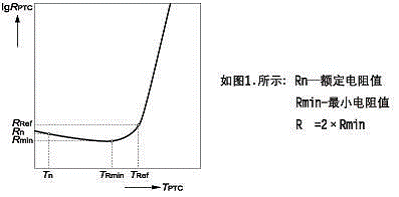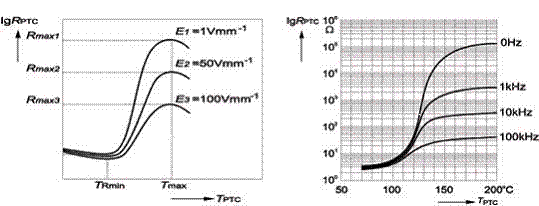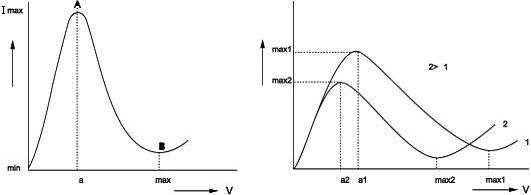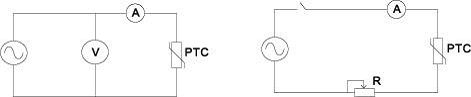﻿ PTCR thermistor several properties analysis_

China sensor manufacturers

# PTCR thermistor several properties analysis

1, PTCR thermistor - temperature characteristics:
Resistance - temperature characteristics is defined in the specified voltage, PTCR thermistor zero resistance and resistance of the relationship between the temperature of the body (Figure 1). Zero power resistance measurement should be carried out in the super thermostatic bath, Usually using pulse voltage, On the pulse power requirements are low output impedance, Output amplitude is stable. Measuring the current caused by PTC thermistor temperature rise,Should be controlled in a negligible range. Figure 2, 3 for the different voltage and frequency resistance-temperature characteristics of the curve, As can be seen from the figure,The resistance at the same temperature, With the test voltage or frequency increases significantly decreased.2, PTCR thermistor - volt-ampere characteristics:

V - Ante sex generally refers to the 25 ℃ in still air, The relationship between the voltage applied to the two terminals of the thermistor and the current under the stable condition to reach the thermal equilibrium. The PTC thermistor in the actual working state of the voltage and current characteristics (Figure 4). Ⅰ is not action or linear region, Ⅱ for the transition zone, Ⅲ for the breakdown zone. When measuring the volt-ampere characteristic curve, Should try to keep the ambient temperature constant, And the current value should be read in the resistance body temperature balance. The measurement circuit shown in Figure 6. Figure 5 for the same PTC thermistor at different temperatures measured volt-ampere characteristic curve.Figure 4. V-amp characteristic curve        Figure 5. V-amp characteristics at different temperaturesFigure 6. Volt-Amp characteristic            7. Current-time characteristic measurement principle
measurement schematic

3, PTCR thermistor - current - time characteristics:
Current-time characteristic refers to the thermistor in the process of applying voltage, The characteristics of the current with time (Figure 8). Current-time characteristics are usually measured using a memory oscilloscope. The basic circuit is shown in Fig. Figure 9 for the action current - action time curve, As can be seen from the figure, The operating time of the PTC thermistor drops abruptly with the increase of the starting current. In addition, the action time and resistance temperature coefficient, The applied voltage is related to the thermal capacity of the thermistor.Figure 8. Current-time characteristics       9. Action current - action time curve

﻿
﻿